SNVS480J January   2007  – July 2020

PRODUCTION DATA.

1. Features
2. Applications
3. Description
1.     Device Images
4. Revision History
5. Pin Configuration and Functions
6. Specifications
7. Detailed Description
1. 7.1 Overview
2. 7.2 Functional Block Diagram
3. 7.3 Feature Description
4. 7.4 Device Functional Modes
8. Application and Implementation
1. 8.1 Application Information
2. 8.2 Typical Application
1. 8.2.1 Design Requirements
2. 8.2.2 Detailed Design Procedure
3. 8.2.3 Application Curves
9. Power Supply Recommendations
10. 10Layout
1. 10.1 Layout Guidelines
2. 10.2 Layout Examples
11. 11Device and Documentation Support
12. 12Mechanical, Packaging, and Orderable Information

• DGS|10

#### 8.2.2.4 Boost Inductor

The first criterion for selecting an inductor is the inductance itself. In fixed-frequency boost converters, this value is based on the desired peak-to-peak ripple current, ΔiL, which flows in the inductor along with the average inductor current, IL. For a boost converter in CCM, IL is greater than the average output current, IO. The two currents are related by Equation 7.

Equation 7. IL = IO / (1 – D)

As with switching frequency, the inductance used is a tradeoff between size and cost. Larger inductance means lower input ripple current, however because the inductor is connected to the output during the off-time only, there is a limit to the reduction in output ripple voltage. Lower inductance results in smaller, less expensive magnetics. An inductance that gives a ripple current of 30% to 50% of IL is a good starting point for a CCM boost converter. Minimum inductance must be calculated with Equation 8 at the extremes of input voltage to find the operating condition with the highest requirement.

Equation 8.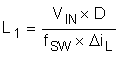By calculating in terms of amperes, volts, and megahertz, the inductance value comes out in micro henries.

To ensure that the boost regulator operates in CCM, a second equation is required, and must also be evaluated with Equation 9 at the corners of input voltage to find the minimum inductance required.

Equation 9.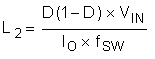By calculating in terms of volts, amps, and megahertz, the inductance value comes out in µH.

For this design, ΔiL is set to 40% of the maximum IL. Duty cycle is evaluated first at VIN(MIN) and at VIN(MAX). Second, the average inductor current is evaluated at the two input voltages. Third, the inductor ripple current is determined. Finally, the inductance can be calculated, and a standard inductor value selected that meets all the criteria.

1. Inductance for Minimum Input Voltage (Equation 10, Equation 11, and Equation 12)
2. Equation 10. DVIN(MIN) = (40 – 9 + 0.5) / (40 + 0.5) = 78% IL-VIN(MIN) = 0.5 / (1 – 0.78) = 2.3 A ΔiL = 0.4 × 2.3 A = 0.92 A
Equation 11.Equation 12.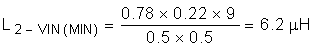3. Inductance for Maximum Input Voltage (Equation 13, Equation 14, and Equation 15)
4. Equation 13. DVIN(MAX) = (40 – 16 + 0.5) / (40 + 0.5) = 60% IL-VIN(MIAX) = 0.5 / (1 – 0.6) = 1.25 A ΔiL = 0.4 × 1.25 A = 0.5 A
Equation 14.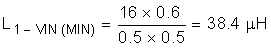Equation 15.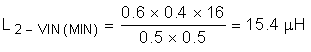Maximum average inductor current occurs at VIN(MIN), and the corresponding inductor ripple current is 0.92 AP-P. Selecting an inductance that exceeds the ripple current requirement at VIN(MIN) and the requirement to stay in CCM for VIN(MAX) provides a tradeoff that allows smaller magnetics at the cost of higher ripple current at maximum input voltage. For this example, a 33-µH inductor satisfies these requirements.

The second criterion for selecting an inductor is the peak current carrying capability. This is the level above which the inductor saturates. In saturation, the inductance can drop off severely, resulting in higher peak current that can overheat the inductor or push the converter into current limit. In a boost converter, peak current, IPK, is equal to the maximum average inductor current plus one half of the ripple current. First, the current ripple must be determined under the conditions that give maximum average inductor current with Equation 16.

Equation 16.Maximum average inductor current occurs at VIN(MIN). Using the selected inductance of 33 µH yields Equation 17.

Equation 17. ΔiL = (9 × 0.78) / (0.5 × 33) = 425 mAP-P

The highest peak inductor current over all operating conditions is therefore Equation 18.

Equation 18. IPK = IL + 0.5 × ΔiL = 2.3 + 0.213 = 2.51 A

Hence, an inductor must be selected that has a peak current rating greater than 2.5 A and an average current rating greater than 2.3 A. One possibility is an off-the-shelf 33 µH ±20% inductor that can handle a peak current of 3.2 A and an average current of 3.4 A. Finally, the inductor current ripple is recalculated with Equation 19 at the maximum input voltage.

Equation 19. ΔiL-VIN(MAX) = (16 × 0.6) / (0.5 × 33) = 0.58 AP-P